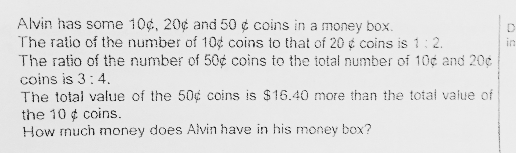# QuestionAnyone help me with attached question

Thanks

 10¢ 20¢ 50¢ 10¢:20¢ = 1:2 1u 2u Multiply by 4 4u 8u 50¢ : 10¢+20¢ = 3 : 4 = 9 : 12 4u 8u 9u

Value of 50¢ coins = 9u × 0.50 = 4.50u

Value of 10¢ coins = 4u  × 0.10 = 0.40u

4.50u – 0.40u = 16.40

4.10u = 16.40

u = 4

Alvin has (16 × 0.10) + (32 × 0.20) + (36 × 0.50) = \$26

10c : 20c : total
1 : 2 : 3
4u : 8u : 12u

50c : 10c + 20c : total
3 : 4 : 7
9u : 12u : 21u

9u x 0.50 = 4.5u ——- 4u x 0.10 + 15.40 = 0.4u + 16.40
1u ——- 16.40/(4.5 – 0.4) = 4
(4 x 4 x 0.10) + (8 x 4 x 0.20) + (9 x 4 x 0.50) = 26

Ans : \$26.

0 Replies 1 Like

36 pieces of 50 cents coins

32 pieces of 20 cents coins

16 pieces of 10 cents coins

in total \$26 in the box

0 Replies 1 Like